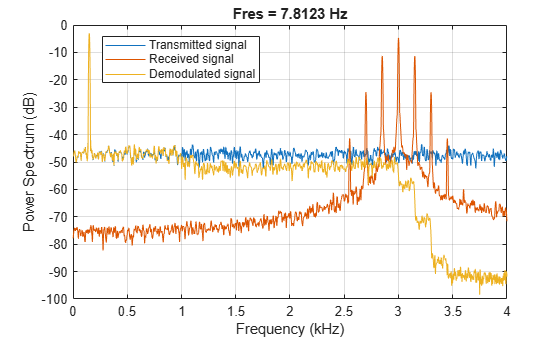# demod

Demodulation for communications simulation

## Syntax

``x = demod(y,fc,fs,method)``
``x = demod(y,fc,fs,method,opt)``

## Description

example

````x = demod(y,fc,fs,method)` demodulates the real carrier signal `y` with a carrier frequency `fc` and sample rate `fs` using the method specified in `method`.```
````x = demod(y,fc,fs,method,opt)` demodulates the real carrier signal `y` using the additional options specified in `opt`.```

## Examples

collapse all

Generate a 150 Hz sinusoid sampled at 8 kHz for 1 second. Embed the modulated signal in white Gaussian noise of variance 0.1².

```fs = 8e3; t = 0:1/fs:1-1/fs; s = cos(2*pi*150*t) + randn(size(t))/10;```

Frequency modulate the signal at a carrier frequency of 3 kHz using a modulation constant of 0.1.

```fc = 3e3; rx = modulate(s,fc,fs,'fm',0.1);```

Frequency demodulate the signal using the same carrier frequency and modulation constant. Compute and plot power spectrum estimates for the transmitted, received, and demodulated signals.

```y = demod(rx,fc,fs,'fm',0.1); pspectrum([s;rx;y]',fs,'Leakage',0.85) legend('Transmitted signal','Received signal','Demodulated signal','Location','best')```## Input Arguments

collapse all

Modulated message signal, specified as a real vector or matrix. Except for the methods `pwm` and `ppm`, `y` is the same size as `x`.

Carrier frequency used to modulate the message signal, specified as a real positive scalar.

Sample rate, specified as a real positive scalar.

Method of modulation used, specified as one of:

• `am` or `amdsb-sc` — Amplitude demodulation, double sideband, suppressed carrier. Multiplies `y` by a sinusoid of frequency `fc` and applies a fifth-order Butterworth lowpass filter using `filtfilt`.

```x = y.*cos(2*pi*fc*t); [b,a] = butter(5,fc*2/fs); x = filtfilt(b,a,x);```
• `amdsb-tc` — Amplitude demodulation, double sideband, transmitted carrier. Multiplies `y` by a sinusoid of frequency `fc` and applies a fifth-order Butterworth lowpass filter using `filtfilt`.

```x = y.*cos(2*pi*fc*t); [b,a] = butter(5,fc*2/fs); x = filtfilt(b,a,x);```

If you specify `opt`, `demod` subtracts scalar `opt` from `x`. The default value for `opt` is 0.

• `amssb` — Amplitude demodulation, single sideband. Multiplies `y` by a sinusoid of frequency `fc` and applies a fifth-order Butterworth lowpass filter using `filtfilt`..

```x = y.*cos(2*pi*fc*t); [b,a] = butter(5,fc*2/fs); x = filtfilt(b,a,x);```
• `fm` — Frequency demodulation. Demodulates the FM waveform by modulating the Hilbert transform of `y` by a complex exponential of frequency `-fc` Hz and obtains the instantaneous frequency of the result..

`y=cos(2*pi*fc*t + opt*cumsum(x))`

`cumsum` is a rectangular approximation of the integral of `x`. `modulate` uses `opt` as the constant of frequency modulation. If you do not specify the `opt` parameter, `modulate` uses a default of `opt = (fc/fs)*2*pi/(max(max(x)))` so the maximum frequency excursion from `fc` is `fc` Hz.

• `pm` — Phase demodulation. Demodulates the PM waveform by modulating the Hilbert transform of `y` by a complex exponential of frequency `-fc` Hz and obtains the instantaneous phase of the result.

`y=cos(2*pi*fc*t + opt*x)`

`modulate` uses `opt` as the constant of phase modulation. If you do not specify the `opt` parameter, `modulate` uses a default of `opt = pi/(max(max(x)))` so the maximum phase excursion is π radians.

• `pwm` — Pulse-width demodulation. Finds the pulse widths of a pulse-width modulated signal `y`. `demod` returns in `x` a vector whose elements specify the width of each pulse in fractions of a period. The pulses in `y` should start at the beginning of each carrier period, that is, they should be left justified. `modulate(x,fc,fs,'pwm','centered')` yields pulses centered at the beginning of each period. The length of `y` is `length(x)*fs/fc`.

• `ppm` — Pulse-position demodulation. Finds the pulse positions of a pulse-position modulated signal `y`. For correct demodulation, the pulses cannot overlap. `x` is length `length(t)*fc/fs`.

• `qam`— Quadrature amplitude demodulation. ```[x1,x2] = demod(y,fc,fs,'qam')``` multiplies `y` by a cosine and a sine of frequency `fc` and applies a fifth-order Butterworth lowpass filter using `filtfilt`.

```x1 = y.*cos(2*pi*fc*t); x2 = y.*sin(2*pi*fc*t); [b,a] = butter(5,fc*2/fs); x1 = filtfilt(b,a,x1); x2 = filtfilt(b,a,x2);```

The input argument `opt` must be the same size as `y`.

Optional input, specified for some methods. Refer to `method` for more details on how to use `opt`.

## Output Arguments

collapse all

Demodulated message signal, returned as a real vector or matrix.

## Version History

Introduced before R2006a